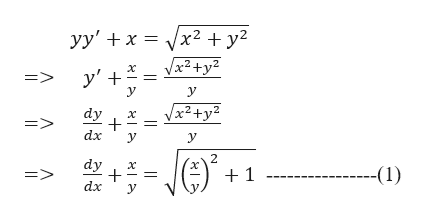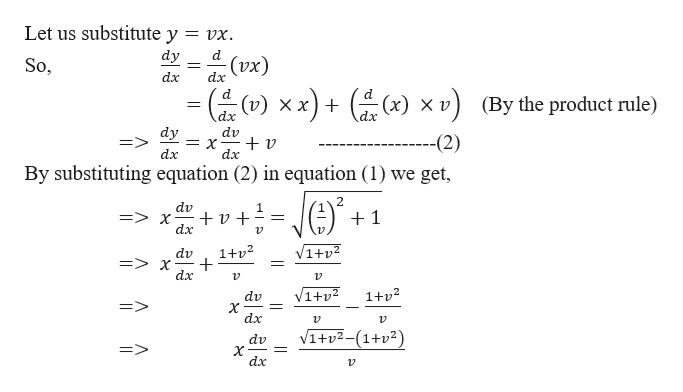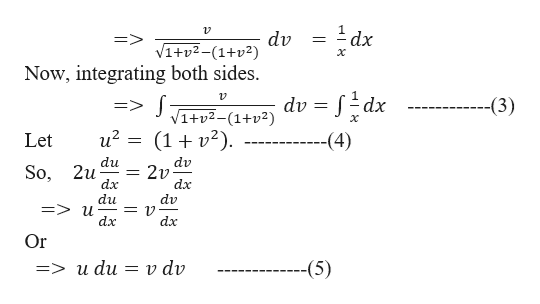# I am super stuck on this problem!:Solve yy'+ x =(x^2+y^2)^1/2I know that I'm supposed to use substitution. I used v=y^2  which v'=2yy' and sub it into the equation. After the substitution, I would get v/2 + x = (x^2+v)^(1/2). After sub, I got stuck in how to separate the v and x with the square root going on. Please help me. Thank you.

Question
1 views

I am super stuck on this problem!:

Solve yy'+ x =(x^2+y^2)^1/2

I know that I'm supposed to use substitution. I used v=y^2  which v'=2yy' and sub it into the equation. After the substitution, I would get v/2 + x = (x^2+v)^(1/2). After sub, I got stuck in how to separate the v and x with the square root going on. Please help me. Thank you.

check_circle

Step 1

We need to solve yy'+ x = (x2+y2)1/2 by the method of substitution.

Let us re-write the given differential equation to visualize the substitution easily.help_outlineImage Transcriptioncloseyy' x2 +y2 Vx2+y2 у + y' y => у Vx2+y2 dy dx У У 2 dy --(1) 1 dx У fullscreen
Step 2

Now,help_outlineImage TranscriptioncloseLet us substitute y = vx dy d (vx) So, dx dx d x) X v (v) X x) + (By the product rule) dx dx dy = X dx dv --(2) dx By substituting equation (2) in equation (1) we get, 2 dv X dx 1 V1+v 1+p2 dv dx 1+p2 V1tv dv х dx t2-(1+2) dv X dx => v fullscreen
Step 3

Solving furt...help_outlineImage Transcriptionclose1 dx 12 dv +v2-(1+2) => х Now, integrating both sides. -Sdx => S dv V1+v2-(1+v2) (3) u2 (12) -(4) Let du So, 2u dx du => u dx dv 2v dx dv = 12 dx Or v dv -(5) 3> и du fullscreen

### Want to see the full answer?

See Solution

#### Want to see this answer and more?

Solutions are written by subject experts who are available 24/7. Questions are typically answered within 1 hour.*

See Solution
*Response times may vary by subject and question.
Tagged in

### Math